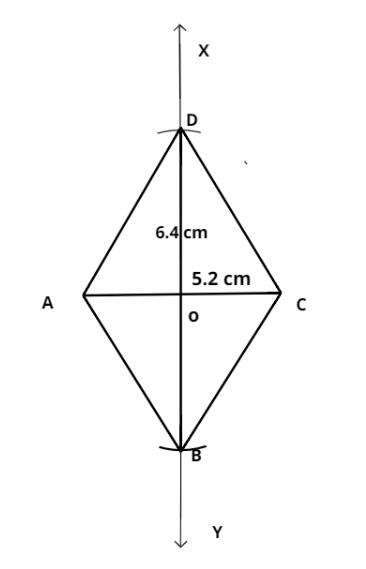Courses
Courses for Kids
Free study material
Free LIVE classes
More# Draw the following:A rhombus whose diagonals are 5.2 cm and 6.4 cm long.

Last updated date: 23rd Mar 2023
Total views: 309.3k
Views today: 7.86kVerified
309.3k+ views
Hint – we use the property of Diagonals of rhombus which bisect perpendicular to each other.
We can draw a rhombus whose diagonals are 5.2 cm and 6.4 cm long by following the given procedure.
1. Draw a line segment AC = 5.2 cm.
2. Draw XY, the perpendicular bisector of AC.
3. From XY, cut-off OD = $\dfrac{1}{2}(6.4)$ = 3.2 cm.
4. Similarly, cut-off OB = $\dfrac{1}{2}(6.4)$= 3.2 cm.
5. Join AD, DCCB and BA.
Thus, ABCD is the required rhombus.Note - In this type of problem, let any cyclic quadrilateral and apply the property of the circle with its circumferences & some geometric properties and we get a rhombus whose diagonals are 5.2 cm and 6.4 cm long.